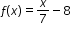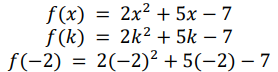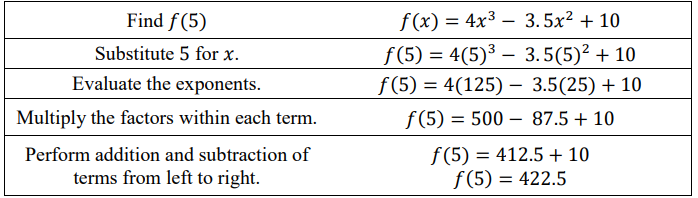# MA.912.F.1.2Export Print
Given a function represented in function notation, evaluate the function for an input in its domain. For a real-world context, interpret the output.

### Examples

Algebra 1 Example: The functionmodels Alicia’s position in miles relative to a water stand x minutes into a marathon. Evaluate and interpret for a quarter of an hour into the race.

### Clarifications

Clarification 1: Problems include simple functions in two-variables, such as f(x,y)=3x-2y.

Clarification 2: Within the Algebra 1 course, functions are limited to one-variable such as f(x)=3x.

General Information
Subject Area: Mathematics (B.E.S.T.)
Strand: Functions
Status: State Board Approved

## Benchmark Instructional Guide

### Terms from the K-12 Glossary

• Function Notation

### Vertical Alignment

Previous Benchmarks

Next Benchmarks

### Purpose and Instructional Strategies

In middle grades, students worked with $x$-$y$ notation and substituted values in expressions and equations. In Algebra I, students work with $x$-$y$ notation and function notation throughout instruction of linear, quadratic, exponential and absolute value functions. In later courses, students will continue to use function notation with other function types and perform operations that combine functions, including compositions of functions.
• Instruction leads students to understand that $f$($x$) reads as “$f$ of $x$” and represents an output of a function equivalent to that of the variable $y$ in $x$-$y$ notation.
• Instruction includes a series of functions with random inputs so that students can see the pattern that emerges (MTR.5.1).
• For example,• Students should discover that the number in parenthesis corresponds to the input or $x$-value on the graph and the number to the right of the equal sign corresponds to the output or $y$-value.
• Although not conventional, instruction includes using function notation flexibly.
• For example, function notation can been seen as $h$($x$) = 4$x$ + 7 or 4$x$ + 7 = $h$($x$).
• Instruction leads students to consider the practicality that function notation presents to mathematicians. In several contexts, multiple functions can exist that we want to consider simultaneously. If each of these functions is written in $x$-$y$ notation, it can lead to confusion in discussions.
• For example, the equations $y$ = −2$x$ + 4 and $y$ = 3$x$ + 7. Representing these functions in function notation allows mathematicians to distinguish them from each other more easily (i.e., $f$($x$) = −2$x$ + 4 and $g$($x$) = 3$x$ + 7).
• Function notation also allows for the use of different symbols for the variables, which can add meaning to the function.
• For example, $h$($t$) = −16$t$2 + 49$t$ + 4 could be used to represent the height, $h$, of a ball in feet over time, $t$, in seconds.
• Function notation allows mathematicians to express the output and input of a function simultaneously.
• For example, $h$(3) = 7 would represent a ball 7 feet in the air after 3 seconds of elapsed time. This is equivalent to the ordered pair (3, 7) but with the added benefit of knowing which function it is associated with.

### Common Misconceptions or Errors

• Throughout students’ prior experience, two variables written next to one another indicate they are being multiplied. That changes in function notation and will likely cause confusion for some of your students. Continue to discuss the meaning of function notation with these students until they become comfortable with the understanding. In other words, $f$($x$) does not mean $f$ · $x$
• Students may need additional support in the order of operations.
• For example, for exponential functions, many students multiply $a$ by the growth factor and then raise the product to the value of the exponent.
• For example, students may think that the multiplication is always performed before division.

### Strategies to Support Tiered Instruction

• Instruction is provided to determine the order of operations required once a given input is placed into the function for evaluation. Students may need additional support determining the correct order of operations to perform.
• Teacher models using parentheses to help organize order of operations when evaluating functions.
• When evaluating $f$($x$) = 4$x$2 for $x$ = −1, teacher can model the use of parentheses by writing the expression 4(−1)2 rather than without using parentheses writing 4 ⋅ −12 This will help students visualize the operations.
•  Teacher provides instruction for identifying the operations in various functions as they relate to the order of operations using a graphic organizer.• The original value of a painting is \$9,000 and the value increases by 7% each year. The value of the painting can be described by the function $V$($t$) = 9000(1 + 0.07)$t$, where $t$ is the time in years since 1984 and $V$($t$) is the value of the painting.
• Part A. Create a table of values that corresponds to this function.
• Part B. Graph the function.

### Instructional Items

Instructional Item 1
• Evaluate $f$(24), when $f$($x$) = $\frac{\text{3}}{\text{2}}$$x$ + 9.

Instructional Item 2
• Given $f$($x$) = 2$x$4 − 0.24 $x$ + 6.17$x$ − 7, find $f$(2).

*The strategies, tasks and items included in the B1G-M are examples and should not be considered comprehensive.

## Related Courses

This benchmark is part of these courses.
1200310: Algebra 1 (Specifically in versions: 2014 - 2015, 2015 - 2022, 2022 and beyond (current))
1200320: Algebra 1 Honors (Specifically in versions: 2014 - 2015, 2015 - 2022, 2022 and beyond (current))
1200370: Algebra 1-A (Specifically in versions: 2014 - 2015, 2015 - 2022, 2022 and beyond (current))
1200380: Algebra 1-B (Specifically in versions: 2014 - 2015, 2015 - 2022, 2022 and beyond (current))
1200400: Foundational Skills in Mathematics 9-12 (Specifically in versions: 2014 - 2015, 2015 - 2022, 2022 and beyond (current))
7912080: Access Algebra 1A (Specifically in versions: 2014 - 2015, 2015 - 2018, 2018 - 2019, 2019 - 2022, 2022 and beyond (current))
7912090: Access Algebra 1B (Specifically in versions: 2014 - 2015, 2015 - 2018, 2018 - 2019, 2019 - 2022, 2022 and beyond (current))
1200315: Algebra 1 for Credit Recovery (Specifically in versions: 2014 - 2015, 2015 - 2022, 2022 and beyond (current))
1200375: Algebra 1-A for Credit Recovery (Specifically in versions: 2014 - 2015, 2015 - 2022, 2022 and beyond (current))
1200385: Algebra 1-B for Credit Recovery (Specifically in versions: 2014 - 2015, 2015 - 2022, 2022 and beyond (current))
7912075: Access Algebra 1 (Specifically in versions: 2014 - 2015, 2015 - 2018, 2018 - 2019, 2019 - 2022, 2022 and beyond (current))
1210305: Mathematics for College Statistics (Specifically in versions: 2022 and beyond (current))
1200388: Mathematics for Data and Financial Literacy Honors (Specifically in versions: 2022 and beyond (current))
1200384: Mathematics for Data and Financial Literacy (Specifically in versions: 2022 and beyond (current))
7912120: Access Mathematics for Data and Financial Literacy (Specifically in versions: 2022 - 2023, 2023 and beyond (current))
1200710: Mathematics for College Algebra (Specifically in versions: 2022 and beyond (current))

## Related Access Points

Alternate version of this benchmark for students with significant cognitive disabilities.
MA.912.F.1.AP.2: Given a function represented in function notation, evaluate the function for an input in its domain.

## Related Resources

Vetted resources educators can use to teach the concepts and skills in this benchmark.

## Formative Assessments

Recursive Sequences:

Students are asked to find the first five terms of a sequence defined recursively, explain why the sequence is a function, and describe its domain

Type: Formative Assessment

Cell Phone Battery Life:

Students are asked to interpret statements that use function notation in the context of a problem.

Type: Formative Assessment

What Is the Value?:

Students are asked to determine the corresponding input value for a given output using a table of values representing a function, f.

Type: Formative Assessment

What Is the Function Notation?:

Students are asked to use function notation to rewrite the formula for the volume of a cube and to explain the meaning of the notation.

Type: Formative Assessment

Graphs and Functions:

Students are asked to determine the value of a function, at an input given using function notation, by inspecting its graph.

Type: Formative Assessment

Evaluating a Function:

Students are asked to evaluate a function at a given value of the independent variable.

Type: Formative Assessment

## Lesson Plans

Select a Healthcare Plan:

Students are asked to determine a procedure for ranking healthcare plans based on their assumptions and the cost of each plan given as a function. Then, they are asked to revise their ranking based on a new set of data.

Type: Lesson Plan

How much is your time worth?:

This lesson is designed to help students solve real-world problems involving compound and continuously compounded interest. Students will also be required to translate word problems into function models, evaluate functions for inputs in their domains, and interpret outputs in context.

Type: Lesson Plan

Freeze:

In this lesson students will learn how to write equations in function notation when given a real-world scenario. Students will work in groups to determine an equation for a given scenario, as well as, write a scenario for a given equation.

Type: Lesson Plan

Turning Tires Model Eliciting Activity:

The Turning Tires MEA provides students with an engineering problem in which they must work as a team to design a procedure to select the best tire material for certain situations. The main focus of the MEA is applying surface area concepts and algebra through modeling.

Type: Lesson Plan

## Original Student Tutorial

Travel with Functions:

Learn how to evaluate and interpret function notation by following Melissa and Jose on their travels in this interactive tutorial.

Type: Original Student Tutorial

## Perspectives Video: Professional/Enthusiast

Hurricane Dennis & Failed Math Models:

What happens when math models go wrong in forecasting hurricanes?

Type: Perspectives Video: Professional/Enthusiast

## STEM Lessons - Model Eliciting Activity

Select a Healthcare Plan:

Students are asked to determine a procedure for ranking healthcare plans based on their assumptions and the cost of each plan given as a function. Then, they are asked to revise their ranking based on a new set of data.

Turning Tires Model Eliciting Activity:

The Turning Tires MEA provides students with an engineering problem in which they must work as a team to design a procedure to select the best tire material for certain situations. The main focus of the MEA is applying surface area concepts and algebra through modeling.

## MFAS Formative Assessments

Cell Phone Battery Life:

Students are asked to interpret statements that use function notation in the context of a problem.

Evaluating a Function:

Students are asked to evaluate a function at a given value of the independent variable.

Graphs and Functions:

Students are asked to determine the value of a function, at an input given using function notation, by inspecting its graph.

Recursive Sequences:

Students are asked to find the first five terms of a sequence defined recursively, explain why the sequence is a function, and describe its domain

What Is the Function Notation?:

Students are asked to use function notation to rewrite the formula for the volume of a cube and to explain the meaning of the notation.

What Is the Value?:

Students are asked to determine the corresponding input value for a given output using a table of values representing a function, f.

## Original Student Tutorials Mathematics - Grades 9-12

Travel with Functions:

Learn how to evaluate and interpret function notation by following Melissa and Jose on their travels in this interactive tutorial.

## Student Resources

Vetted resources students can use to learn the concepts and skills in this benchmark.

## Original Student Tutorial

Travel with Functions:

Learn how to evaluate and interpret function notation by following Melissa and Jose on their travels in this interactive tutorial.

Type: Original Student Tutorial

## Parent Resources

Vetted resources caregivers can use to help students learn the concepts and skills in this benchmark.